# Equation + system of equations - math problems

#### Number of problems found: 502

• Null points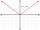Calculate the roots of the equation: ?
• Non linear eqsSolve the system of non-linear equations: 3x2-3x-y=-2 -6x2-x-y=-7
• Substitutionsolve equations by substitution: x+y= 11 y=5x-25
• Hyperbola equationFind the hyperbola equation with the center of S [0; 0], passing through the points: A [5; 3] B [8; -10]
• Parabola 3Find the equation of a parabola with its focus at (0,2) and its vertex at the origin. ?
• ParabolaFind the equation of a parabola that contains the points at A[6; -5], B[14; 9], C[23; 6]. (use y = ax2+bx+c)
• Touch x-axisFind the equations of circles that pass through points A (-2; 4) and B (0; 2) and touch the x-axis.
• Find the 13Find the equation of the circle inscribed in the rhombus ABCD where A[1, -2], B[8, -3] and C[9, 4].Write the equation of the quadratic function which includes points A (-1, 10), B (2, 19), C (1,4)
• There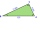There is a triangle ABC: A (-2,3), B (4, -1), C (2,5). Determine the general equations of the lines on which they lie: a) AB side, b) height to side c, c) Axis of the AB side, d) median ta to side a
• LineWrite an equation of a line parallel to To 9x + 3y = 8 That Passes Through The Point (-1, -4). Write in form ax+by=c.
• Geometric sequence 5About members of geometric sequence we know: ? ? Calculate a1 (first member) and q (common ratio or q-coefficient)
• Linear function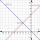What is the equation of linear function passing through points: a) A (0,3), B (3,0) b) A (-2,-6), B (3,4)
• Find d 2Find d in an A. P. whose 5th term is 18 and 39th term is 120.
• Product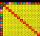The sum and the product of three integers is 6. Write largest of them.
• The ratioThe ratio of two numbers is 4, their sum is 75. Determine both numbers.
• Have solutionThe sum of four consecutive even numbers is 92. Determine these numbers.
• Algebrogram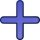Solve algebrogram: mama+anna=vari How many solutions are?
• Geometric sequenceIn the geometric sequence is a4 = 20 a9 = -160. Calculate the first member a1 and quotient q.
• Internal angles IST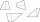Determine internal angles of isosceles trapezium ABCD /a, c are the bases/ and if: alpha:gamma = 1:3

Do you have an interesting mathematical word problem that you can't solve it? Submit a math problem, and we can try to solve it.

We will send a solution to your e-mail address. Solved examples are also published here. Please enter the e-mail correctly and check whether you don't have a full mailbox.

Please do not submit problems from current active competitions such as Mathematical Olympiad, correspondence seminars etc...

Do you have a system of equations and looking for calculator system of linear equations? Do you have a linear equation or system of equations and looking for its solution? Or do you have quadratic equation? Equations Math problems. System of equations - math word problems.